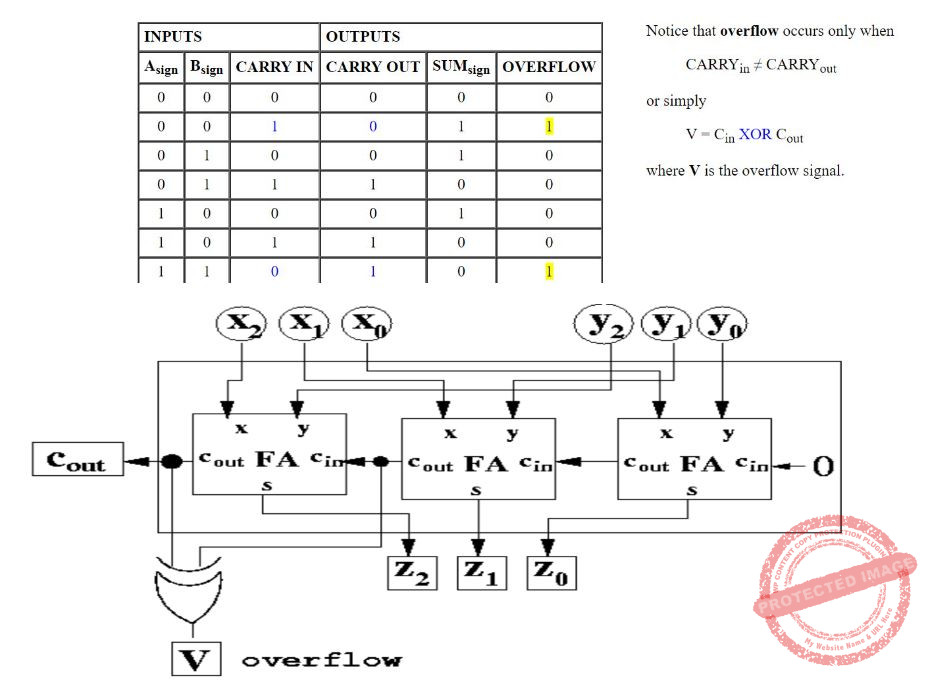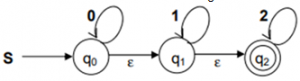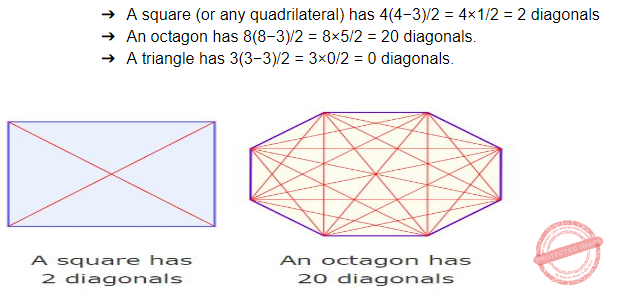## ISRO CS 2013

 Question 1
In graphics, the number of vanishing points depends on
 A the number of axes cut by the projection plane B the centre of projection C the number of axes which are parallel to the projection plane D the perspective projections of any set of parallel lines that are not parallel to the projection plane
Graphics       Vanishing-pointer       Video-Explanation
Question 1 Explanation:
→Projections of lines that are not parallel to the view plane (i.e. lines that are not perpendicular to the view plane normal) appear to meet at some point on the view plane.
→This point is called the vanishing point. A vanishing point corresponds to every set of parallel lines.
 Question 2
The built-in base class in Java, which is used to handle all exceptions is
 A Raise B Exception C Error D Throwable
Java       Properties       Video-Explanation
Question 2 Explanation:
Throwable class is the built-in base class used to handle all the exceptions in Java.
 Question 3
Opportunistic reasoning is addressed by which of the following knowledge representation
 A Script B Blackboard C Production Rules D Fuzzy Logic
Research-aptitude       Knowledge-representation       Video-Explanation
Question 3 Explanation:
→ Opportunistic reasoning:
It is a method of selecting a suitable logical inference strategy within artificial intelligence applications.
Opportunistic reasoning has been used in applications such as blackboard systems and medical applications.
→ Specific reasoning methods may be used to draw conclusions from a set of given facts in a knowledge base, e.g. forward chaining versus backward chaining.
→ However, in opportunistic reasoning, pieces of knowledge may be applied either forward or backward, at the "most opportune time".
→ An opportunistic reasoning system may combine elements of both forward and backward reasoning.
→ It is useful when the number of possible inferences is very large and the reasoning system must be responsive to new data that may become known.
 Question 4
The following steps in a linked list
p = getnode()
info (p) = 10
next (p) = list
list = p
result in which type of operation?
 A pop operation in stack B removal of a node C inserting a node D modifying an existing node
Data-Structures       Programming       Video-Explanation
Question 4 Explanation:
p = getnode() // Allocating memory for node and starting address of that node will store in the pointer “p”
info (p) = 10 // Storing the value of 10 into the info field of new node
next (p) = list // adding new node to the existing list.
list=p // the starting address of the list will
point to the new node
 Question 5
Shift reduce parsing belongs to a class of
 A bottom up parsing B top down parsing C recursive parsing D predictive parsing
Compiler-Design       Parsers       Video-Explanation
Question 5 Explanation:
→ A shift-reduce parser is a class of efficient, table-driven bottom-up parsing methods for computer languages and other notations formally defined by a grammar.
→ The parsing methods most commonly used for parsing programming languages, LR parsing and its variations, are shift-reduce methods.Question 6
Which of the following is not provided as a service in cloud computing?
 A Infrastructure as a service B Architecture as a service C Software as a service D Platform as a service
Cloud-Computing       Cloud-services       Video-Explanation
Question 6 Explanation:
→Cloud computing is the delivery of computing services—servers, storage, databases, networking, software, analytics, intelligence and more—over the Internet (“the cloud”) to offer faster innovation, flexible resources and economies of scale.
→Most cloud computing services fall into four broad categories: infrastructure as a service (IaaS), platform as a service (PaaS), serverless and software as a service (SaaS).
 Question 7
The binary equivalent of the decimal number 42.75 is
 A 101010 B 100110 C 101010 D 100110
Digital-Logic-Design       Number-system       Video-Explanation
Question 7 Explanation:
(42.75)10 = (?)2Question 8
Which logic gate is used to detect overflow in 2’s complement arithmetic?
 A OR gate B AND gate C NAND gate D XOR gate
Digital-Logic-Design       Boolean-algebra       Video-Explanation
Question 8 Explanation:Question 9
The number of edges in a ‘n’ vertex complete graph is ?
 A n * (n-1) / 2 B n * (n+1) / 2 C n2 D n * (n+1)
Engineering-Mathematics       Graph-theory       Video-Explanation
Question 9 Explanation:
Complete graph is an undirected graph in which each vertex is connected to every vertex, other than itself. If there are ‘n’ vertices then total edges in a complete graph is n(n-1)/2
 Question 10
What is the right way to declare a copy constructor of a class if the name of the class is MyClass?
 A MyClass (constant MyClass *arg) B MyClass (constant MyClass &arg) C MyClass (MyClass arg) D MyClass (MyClass *arg)
Java       Class-function       Video-Explanation
Question 10 Explanation:
→Copy Constructor is a type of constructor which is used to create a copy of an already existing object of a class type.
→Declaration of copy constructor syntax is different from c++ to java.
→In C++, It is usually of the form class-name (Class_name &vn),
→In Java, the syntax is Class_name(class_name vn).
→So w.r.to C++ , option (B) is correct and w.r.to Java option (c ) is correct.
 Question 11
When two BCD numbers 0x14 and 0x08 are added what is the binary representation of the resultant number?
 A 0x22 B 0x1c C 0x16 D results in overflow
Digital-Logic-Design       Number-system       Video-Explanation
Question 11 Explanation:
In the BCD numbering system, a decimal number is separated into four bits for each decimal digit within the number. Each decimal digit is represented by its weighted binary value performing a direct translation of the number. So a 4-bit group represents each displayed decimal digit from 0000 for a zero to 1001 for a nine
Representation of the above two numbers in the 4-bit number format as follows
0x14 = 0001 0100
0x08 = 0000 1000
1. After performing the addition of the two binary numbers , we will get 0001 110
2. The lower value of BCD is 0000 which is 0 and upper value is1001 which is 9 If the four bit result of addition is greater than 9 and if a carry bit is present in the result then it is invalid and we have to add 6 whose binary equivalent is (0110)2 to the result of addition. Then the resultant that we would get will be a valid binary coded number
The result from the BCD addition is greater than 9,So we need to add to “6” to the result.
0001 1100
0000 0110
--------------
0010 0010 (22)
So, the final resultant number is 22
 Question 12
Which of the following sorting algorithms has the minimum running time complexity in the best and average case?
 A Insertion sort, Quick sort B Quick sort, Quick sort C Quick sort, Insertion sort D Insertion sort, Insertion sort
Algorithms       Sorting       Video-Explanation
Question 12 Explanation:Question 13
The number 1102 in base 3 is equivalent to 123 in which base system?
 A 4 B 5 C 6 D 8
Digital-Logic-Design       Number-system       Video-Explanation
Question 13 Explanation:
Let us consider the base be ‘x’.
(1102)3 = (123) x
= 1x3x3x3 + 1x3x3 + 2 = 1x2 + 2x + 3
= 27 + 9 + 2 = 1x2 + 2x + 3
= x = 5
 Question 14
The number of elements in the power set of the set {{A,B},C} is
 A 7 B 8 C 3 D 4
Engineering-Mathematics       Set-theory       Video-Explanation
Question 14 Explanation:
For ‘n’ elements in a set, there are 2n elements in the corresponding power set.
Given set = {{A, B},C}
Power set = { {{A, B}}, {C}, {{A, B}, C}}, ϕ}
So, total 4 elements are present in the power set.
 Question 15
What are the final states of the DFA generated from the following NFA?A q0, q1, q2 B [q0, q1], [q0, q2], [ ] C q0, [q1, q2] D [q0, q1], q2
Theory-of-Computation       DFA       Video-Explanation
Question 15 Explanation:
→An epsilon transition allows an automaton to change its state spontaneously, i.e. without consuming an input symbol.
→From the diagram, initially “q2” is final state.
→As there is epsilon transitions from qo to q1 and q1 to q2 and finally we reach the end state so q0,q1 are part of final states then q0,q1 and q2 are final states.
 Question 16
How many diagonals can be drawn by joining the angular points of an octagon?How many diagonals can be drawn by joining the angular points of an octagon?
 A 14 B 20 C 21 D 28
Aptitude       Geometry       Video-Explanation
Question 16 Explanation:
→A polygon's diagonals are line segments from one corner to another (but not the edges).
→The number of diagonals of an n-sided polygon is: n(n − 3) / 2.
→Examples:Question 17
Let P(E) denote the probability of the occurrence of event E. If P(A) = 0.5 and P(B) = 1, then the values of P(A/B) and P(B/A) respectively are
 A 0.5, 0.25 B 0.25, 0.5 C 0.5, 1 D 1, 0.5
Engineering-Mathematics       Probability       Video-Explanation
Question 17 Explanation:
Given data is
→P(E) denote the probability of the occurrence of event E
→P(A) = 0.5 and P(B) = 1
→Conditional probability is a measure of the probability of an event (some particular situation occurring) given that another event has occurred.
→If the event of interest is A and the event B is known or assumed to have occurred, "the conditional probability of A given B", or "the probability of A under the condition B", is usually written as P(A | B), or PB(A)
→P(A/B) = P(A ∩ B)/P(B)
→If two events A and B are independent, then the probability of both events is the product of the probabilities for each event: P(A ∩ B) = P(A)P(B)
→P(A/B) = P(A) * P(B) / P(B)
→P(A/B) = 0.5
→Similarly, P(B/A) = P(A) * P(B) / P(A) and P(B/A) = 1
 Question 18
Which of the following strategy is employed for overcoming the priority inversion problem?
 A Temporarily raise the priority of lower priority level process B Have a fixed priority level scheme C Implement kernel preemption scheme D Allow lower priority process to complete its job
Question 18 Explanation:
Priority inversion is the condition in which a high priority task needs to wait for a low priority task to release a resource between the medium priority task and a low priority task.
 Question 19
What is the maximum number of characters (7 bits + parity ) that can be transmitted in a second on a 19.2 kbps line? This asynchronous transmission requires 1 start bit and 1 stop bit.
 A 192 B 240 C 1920 D 1966
Computer-Networks       Serial-communication       Video-Explanation
Question 19 Explanation:
In asynchronous transmission mode, start bit and and stop bit is always required whereas in synchronous mode these bits are not required.
In the given question asynchronous transmission is mentioned and number of bits required is 10 bits. (7 data bits + 1 parity bit + 1 start bit + 1 stop bit )
Bandwidth = 19.2 kbps
Maximum number of characters transmitted in 1 second = (19.2 *1000)/10 = 1920
 Question 20
IEEE 1394 is related to
 A RS-232 B USB C Firewire D PCI
Computer-Networks       IEEE       Video-Explanation
Question 20 Explanation:
→ IEEE 1394 is an interface standard for a serial bus for high-speed communications and isochronous real-time data transfer.
→ It was developed in the late 1980s and early 1990s by Apple, which called it FireWire.
→ The copper cable it uses in its most common implementation can be up to 4.5 metres (15 ft) long. Power is also carried over this cable, allowing devices with moderate power requirements to operate without a separate power supply.
→ FireWire is also available in Cat 5 and optical fiber versions.
→ The 1394 interface is comparable to USB, though USB requires a master controller and has greater market share
There are 20 questions to complete.

Register Now